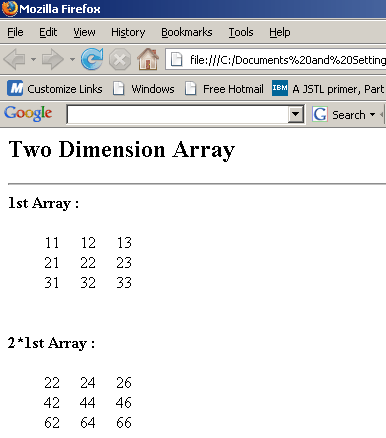# JavaScript array dimension

Tutorials# JavaScript array dimension

In this code we help you to understand array dimension. In this code we use Java Script as scripting language.

In this code we help you to understand array dimension. In this code we use Java Script as scripting language.

# JavaScript array dimension

In this code we help you to understand array dimension. In this code we use  Java Script as scripting language. The code shows you to make a Two dimension array. The page on loading invoke a function display ( ) that include an array variable used to instantiate an array object with initial size of 3.The for loop run and execute the script till the length  of row, column i.e i,j is less than 3 .The document. write print an array with three rows and columns. Again the for loop run and execute for the element in an array till the length i,j is less than 3.Finally the document. write print the element in a 1st array.

The same procedure is implied to print 2nd array dimension. In this array we print the twice of element in first array using document. write.

Array Dimension.html
 ```     Array Dimension                      ```

Output